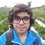# Pre-RMO 2014/1

A natural number $k$ is such that $k^2 < 2014 < (k + 1)^2.$ What is the largest prime factor of $k$?

This note is part of the set Pre-RMO 2014Note by Pranshu Gaba
6 years, 9 months ago

This discussion board is a place to discuss our Daily Challenges and the math and science related to those challenges. Explanations are more than just a solution — they should explain the steps and thinking strategies that you used to obtain the solution. Comments should further the discussion of math and science.

When posting on Brilliant:

• Use the emojis to react to an explanation, whether you're congratulating a job well done , or just really confused .
• Ask specific questions about the challenge or the steps in somebody's explanation. Well-posed questions can add a lot to the discussion, but posting "I don't understand!" doesn't help anyone.
• Try to contribute something new to the discussion, whether it is an extension, generalization or other idea related to the challenge.

MarkdownAppears as
*italics* or _italics_ italics
**bold** or __bold__ bold
- bulleted- list
• bulleted
• list
1. numbered2. list
1. numbered
2. list
Note: you must add a full line of space before and after lists for them to show up correctly
paragraph 1paragraph 2

paragraph 1

paragraph 2

[example link](https://brilliant.org)example link
> This is a quote
This is a quote
    # I indented these lines
# 4 spaces, and now they show
# up as a code block.

print "hello world"
# I indented these lines
# 4 spaces, and now they show
# up as a code block.

print "hello world"
MathAppears as
Remember to wrap math in $$ ... $$ or $ ... $ to ensure proper formatting.
2 \times 3 $2 \times 3$
2^{34} $2^{34}$
a_{i-1} $a_{i-1}$
\frac{2}{3} $\frac{2}{3}$
\sqrt{2} $\sqrt{2}$
\sum_{i=1}^3 $\sum_{i=1}^3$
\sin \theta $\sin \theta$
\boxed{123} $\boxed{123}$

Sort by:

$44^2 < 2014 < (44+1)^2$. This satisfies the above inequality. Therefore, $k = 44$. The largest prime factor of 44 is 11. 11 is the answer.

- 6 years, 9 months ago

To find Largest prime factor of any number K , you need to do its prime factorisation. for e.g.

prime factorisation of 10 is 10=5*2

so the largest prime factor is 10

But the question you asked is something like K^2<2014<(K+1)^2 if we take K^2<2014

we get k<+-44.88….. so largest value of K is 44

we take largest value so we can get largest prime factor. prime factorisation of 44 is -

44= 2211

therefore largest prime factor of K is 11.

- 4 years, 1 month ago

11 is the answer as the valuebof k is 44. Prime factorization of 44= 2 \times 2 \times 11

- 6 years, 9 months ago

11

- 6 years, 9 months ago

<=> 0 < 2014 - k^2 < 2k +1 taking the right side of the inequallity and solving with the restrictions we get that the only integer is 44=4*11 therefore the answer is 11

- 6 years, 9 months ago

k^2<2014. Max. value integer value of 'k' is 44 & it also satisfy the second equation i.e. (k+1)^2 >2014 . Hence largest value of k is 44 & its biggest prime factor is 11. So answer is 11.

- 6 years, 9 months ago

2014 lies between 44 and 45 square. But 45 square is 2025. Therefore we take 44. The prime factors of 44 are 2 and 11. The largest prime factor is 11 and smallest prime factor is 2.

- 6 years, 9 months ago

11

- 6 years, 9 months ago

11

- 6 years, 9 months ago

Here k=44 satisfies the inequality.Now the prime factors of k are 2 and 11, of which 11 is the largest prime factor.

- 6 years, 9 months ago

11

- 6 years, 9 months ago

11

- 6 years, 9 months ago

11

- 6 years, 9 months ago

k2<2o14 ..k<√2014 ...k<44.8 ..also ...2014<k2+2k+1 ...2014,(k+1)2 ...√2014<(k+1) ...44.8<(k+1) ...43.8<k ...k=44

- 6 years, 9 months ago

11

- 6 years, 9 months ago

- 6 years, 9 months ago

${44}^{2}$=1936<2014<${45}^{2}$=2025,so if k <44 $({k}+{1})^{2}$<2014 therefore k=44 so the largest prime factor of k is 11.

- 6 years, 8 months ago

11

- 6 years, 8 months ago

11

- 6 years, 1 month ago

Easy....11

- 5 years, 11 months ago

11

- 5 years, 11 months ago

11

- 5 years, 11 months ago